Courses

# NCERT Exemplar - Some p-Block Elements JEE Notes | EduRev

## JEE : NCERT Exemplar - Some p-Block Elements JEE Notes | EduRev

The document NCERT Exemplar - Some p-Block Elements JEE Notes | EduRev is a part of the JEE Course NEET Revision Notes.
All you need of JEE at this link: JEE

MULTIPLE CHOICE QUESTIONS - I

Q.1. The element which exists in liquid state for a wide range of temperature and can be used for measuring high temperature is
(1) B
(2) Al
(3) Ga
(4) In
Ans. (3) Ga
Solution.

Gallium has different structure consisting Ga2 molecule with lowest melting point. It exists as a liquid from 30°C to 2000°C and hence it is used in high temperature thermometry.

Q.2. Which of the following is a Lewis acid?
(1) AlCl3
(2) MgCl2
(3) CaCl2
(4) BaCl2
Ans.
(1) AlCl3
Solution.

Lewis acids are the species in which octate is not complete and ready to accept electrons. In AlCl3, Al is surrounded by 6 electrons and all three Cl atoms are surrounded by 8 electrons, therefore, AlCl3 is electron accepter. It is a covalent compound.

Q.3. The geometry of a complex species can be understood from the knowledge of type of hybridisation of orbitals of central atom. The hybridisation of orbitals of central atom in [Be(OH)4] and the geometry of the complex  are respectively
(1) sp3 , tetrahedral
(2) sp3 , square planar
(3) spd2 , octahedral
(4) dsp2 , square planar
Ans.
(1) sp3, tetrahedral
Solution.
In[B(OH)4]-, Boron is surrounded by 4 bonds pair and has no lone pair. Geometry and hybridization of central atom is based on bond pairs and lone pairs around the central atom. [B(OH)4]- is tetrahedral and Boron is sp3 hybridized.

Q.4. Which of the following oxides is acidic in nature?
(1) B2O3
(2) Al2O3
(3) Ga2O3
(4) In2O3
Ans.
(1) B2O3
Solution.

In group 13 (boron family) on moving down the group, acidic character decreases and basic character increases, therefore, B2O3 is acidic in nature.

Q.5. The exhibition of highest co-ordination number depends on the availability of vacant orbitals in the central atom. Which of the following elements is not likely to act as central atom in MF63– ?
(1) B
(2) Al
(3) Ga
(4) In

Ans. (1) B
Solution.
Boron does not have d-orbital. The element M in the complex ion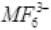has coordination number 6. Boron can have maximum coordination number 4. Thus, B cannot form this complex.

Q.6. Boric acid is an acid because its molecule
(1) Contains replaceable H+ ion
(2) Gives up a proton
(3) Accepts OH from water releasing proton
(4) Combines with proton from water molecule
Ans.
(3) Accepts OH from water releasing proton
Solution.

Boric acid is Lewis acid and accepts electron. It reacts with water and accepts OH- and release H+ ion and thus, acts as weak monobasic acid.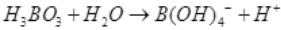Q.7. Catenation i.e., linking of similar atoms depends on size and electronic configuration of atoms. The tendency of catenation in Group 14 elements follows the order:
(1) C > Si > Ge > Sn
(2) C >> Si > Ge ≈ Sn
(3) Si > C > Sn > Ge
(4) Ge > Sn > Si > C
Ans.
(2) C >> Si > Ge ≈ Sn
Solution.
On moving down the group the size of the atom increases and the bond energy decreases and property of catenation decreases. In group 14 carbon shows maximum catenation. C>> Si > Ge ≈ Sn.

Q.8. Silicon has a strong tendency to form polymers like silicones. The chain length of silicone polymer can be controlled by adding
(1) MeSiCl3
(2) Me2SiCl2
(3) Me3SiCl
(4) Me4Si
Ans.
(3) Me3SiCl
Solution
.
The chain length of the polymer can be controlled by adding (CH3)3SiCl  which blocks the ends.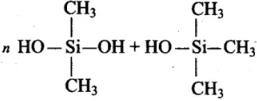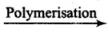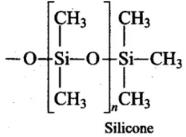Q.9. Ionisation enthalpy (∆iH1 kJ mol–1) for the elements of Group 13 follows the order.
(1) B > Al > Ga > In > Tl
(2) B < Al < Ga < In < Tl
(3) B < Al > Ga < In > Tl
(4) B > Al < Ga > In < Tl

Ans. (4)
Solution.
On moving down the group from B to Tl, a regular decreasing trend in the ionisation energy values is not observed.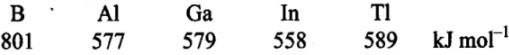In Ga, there are ten d-electrons in the penultimate shell which screen the nuclear charge less effectively and thus, outer electron is held firmly. As a result, the ionisation energy of both A1 and Ga is nearly the same. The increase in ionisation energy from In to Tl is due to poor screening effect of 14f electrons present in the inner shell.

Q.10. In the structure of diborane
(1) All hydrogen atoms lie in one plane and boron atoms lie in a plane perpendicular to this plane.
(2) 2 boron atoms and 4 terminal hydrogen atoms lie in the same plane and 2 bridging hydrogen atoms lie in the perpendicular plane.
(3) 4 bridging hydrogen atoms and boron atoms lie in one plane and two terminal hydrogen atoms lie in a plane perpendicular to this plane.
(4) All the atoms are in the same plane.
Ans.
(2)
Solution.

Four terminal hydrogen atoms and two boron atoms lie in the same plane and two hydrogen atoms forming bridges lie in a plane perpendicular to the rest of the molecule.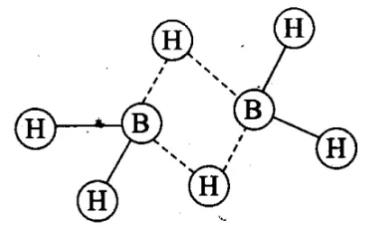Q.11. A compound X, of boron reacts with NH3 on heating to give another compound Y which is called inorganic benzene. The compound X can be prepared by treating BF3 with Lithium aluminium hydride. The compounds X and Y are represented by the formulas.
(1) B2H6 , B3N3H6
(2) B2O3, B3N3H6
(3) BF3, B3N3H6
(4) B3N3H6 , B2H6
Ans.
(1)
Solution.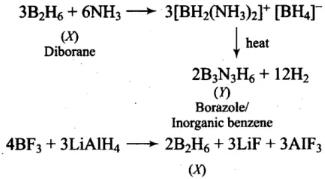Q.12. Quartz is extensively used as a piezoelectric material, it contains ___________.
(1) Pb
(2) Si
(3) Ti
(4) Sn
Ans.
(2)
Solution.
Quartz is a crystalline form of silica.

Q.13. The most commonly used reducing agent is
(1) AlCl3
(2) PbCl2
(3) SnCl4
(4) SnCl2
Ans.
(4)
Solution.

+4 oxidation state of Sn is more stable than +2 oxidation state. Therefore, Sn2+ can be easily oxidised to Sn4+ and hence SnCl2 acts a reducing agent.
SnCl2 + 2Cl → SnCl4 + 2e

Q.14. Dry ice is
(1) Solid NH3
(2) Solid SO2
(3) Solid CO2
(4) Solid N2
Ans.
(3)
Solution.
Solid C02 is known as dry ice.

Q.15. Cement, the important building material is a mixture of oxides of several elements. Besides calcium, iron and sulphur, oxides of elements of which of the group(s) are present in the mixture?
(1) Group 2
(2) Groups 2, 13 and 14
(3) Groups 2 and 13
(4) Groups 2 and 14
Ans.
(2)
Solution.

Cement contains elements of group 2 (Ca), group 13 (Al) and group 14 (Si).

MULTIPLE CHOICE QUESTIONS -II

In the following questions two or more options may be correct.
Q.16. The reason for small radius of Ga compared to Al is _______.
(1) Poor screening effect of d and f orbitals
(2) Increase in nuclear charge
(3) Presence of higher orbitals
(4) Higher atomic number
Ans. (1, 2)

Solution.
The additional 10 d-electrons offer poor screening effect for the outer electrons from the increased fluclear charge in Gallium. Hence, atomic radius of Gallium is less than that of aluminium.

Q.17. The linear shape of CO2 is due to _________.
(1) sp3 hybridisation of carbon
(2) sp hybridisation of carbon
(3) pπ – pπ bonding between carbon and oxygen
(4) sp2 hybridisation of carbon
Ans.
(2, 3)
Solution.

The linear shape of CO2 is due to pπ-pπ bonding between carbon and oxygen and sp hybridisation of carbon.

Q.18. Me3SiCl is used during polymerisation of organo silicones because
(1) the chain length of organo silicone polymers can be controlled by adding Me3SiCl.
(2) Me3SiCl blocks the end terminal of silicone polymer.
(3) Me3SiCl improves the quality and yield of the polymer.
(4) Me3Si Cl acts as a catalyst during polymerisation.
Ans.
(1, 2)
Solution.

The chain length of the polymer can be controlled by adding Me3SiCl which blocks the ends of the silicon polymer.

Q.19. Which of the following statements are correct?
(1) Fullerenes have dangling bonds
(2) Fullerenes are cage-like molecules
(3) Graphite is thermodynamically most stable allotrope of carbon
(4) Graphite is slippery and hard and therefore used as a dry lubricant in machines
Ans.
(2, 3)
Solution.

Fullerenes are cage-like (soccer or rugby ball) molecules and graphite is thermodynamically most stable allotrope of carbon. Thus, options (b) and (c) are correct.

Q.20. Which of the following statements are correct. Answer on the basis of Fig.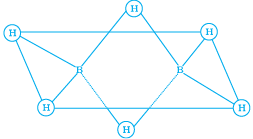(1) The two bridged hydrogen atoms and the two boron atoms lie in one plane.
(2) Out of six B – H, bonds two bonds can be described in terms of 3 centre 2-electron bonds.
(3) Out of six B – H bonds four B – H bonds can be described in terms of 3 centre 2 electron bonds.
(4) The four terminal B – H bonds are two centre-two electron regular bonds.
Ans. (1, 2, 3)
Solution.
Each of the two boron atoms is in sp3-hybrid state. Of the four hybrid orbitals, three have one electron each while the fourth is empty. Two of the four orbitals of each, of the boron atom overlap with two terminal hydrogen atoms forming two normal B – H σ - bonds. One of the remaining hybrid orbitals (either empty or singly occupied) of one of the boron atoms, 15-orbital of H (bridge atom) and one of hybrid orbitals of the other boron atom overlap to form a delocalized orbital covering the three nuclei with a pair of electrons. This is three centre two electron bond. Similar overlapping occurs with the second hydrogen atom (bridging) forming three centre two electrons bond.

Q.21. Identify the correct resonance structures of carbon dioxide from the ones given below :
(1) O – C ≡ O
(2) O = C = O
(3) O ≡ C – O+
(4) –O – C ≡ O+
Ans.
(ii), (iv)
Solution.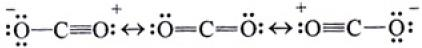Resonance explains delocalized electrons within certain molecules or polyatomic ions where the bonding cannot be expressed by one single Lewis formula.

SHORT ANSWER TYPE QUESTIONS

Q.22. Draw the structures of BCl3.NH3 and AlCl3 (dimer).
Ans. In BCl3, the central B atom has six electrons in the valence shell. It is, therefore, an electron deficient molecule and needs two more electrons to ‘ complete its octet. In other words, BCl3 acts as a Lewis acid. NH3, on the other hand, has a lone pair of electrons which it can donate easily. Therefore, NH3 acts as a Lewis base. The Lewis acid (BC13) and the Lewis base (NH3) combine together to form an adduct as shown below: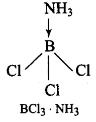In A1C13, A1 has six electrons in the valence shell. Therefore, it is an electron deficient molecule and needs two more electrons to complete its octet.
Chlorine, on the other hand, has three lone pairs of electrons. Therefore, to complete its octet, the central A1 atom of one molecule accepts a lone pair of electrons from Cl atom of the other molecule forming a dimeric structure as shown below.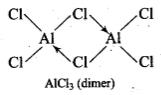Q.23. Explain the nature of boric acid as a Lewis acid in water.
Ans.
Boric acid acts as Lewis acid in water by accepting a pair of electrons from a hydroxyl ion:
B(OH)3 + 2H2O →[B(OH)4] + H3O+

Q.24. Draw the structure of boric acid showing hydrogen bonding. Which species is present in water? What is the hybridisation of boron in this species?
Ans
.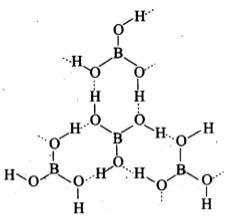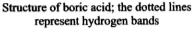[B(OH)4] units are present in water. Boron has sp3 hybridisation in [B(OH)4] unit.

Q.25. Explain why the following compounds behave as Lewis acids?
(1) BCl3
(2) AlCl3

Ans. (1) BCl3: Boron has 6 electrons in its outermost orbital and has a vacant p orbital. Thus, it is an electron deficient compound. Hence, it acts as Lewis acid and accepts a lone pair of electrons.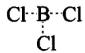(2) AlCl3 is also an electron deficient compound and acts as Lewis acid. It generally forms a dimer to achieve stability.

Q.26. Give reasons for the following:
(1) CCl4 is immiscible in water, whereas SiCl4 is easily hydrolysed.
(2) Carbon has a strong tendency for catenation compared to silicon.
Ans. (1) CC14 is a covalent compound while H2O is a polar compound. Therefore, it is insoluble in water. Alternatively, CCl4 is insoluble in water because carbon does not have (/-orbitals to accommodate the electrons donated by oxygen atom of water molecules. As a result, there is no interaction between CCl4 and water molecules and hence CCl4 is insoluble in water. On the other hand, SiCl4 has d-orbitals to accommodate the lone pair of electrons donated by oxygen atom of water molecules. As a result, there is a strong interaction between SiCl4 and water molecules. Consequently, SiCl4 undergoes hydrolysis by water to form silicic acid.
(2)  The bond dissociation energy decreases rapidly as the atomic size increases. Since the atomic size of carbon is much smaller (77 pm) as compared to that of silicon (118 pm), therefore, carbon-carbon bond dissociation energy is much higher (348 kJ mol-1) than that of silicon-silicon bond (297 kJ mol-1). Hence, because C – C bonds are much stronger as compared to Si-Si bonds, carbon has a much higher tendency for catenation than silicon.

Q.27. Explain the following :
(1) CO2 is a gas whereas SiO2 is a solid.
(2) Silicon forms SiF62– ion whereas corresponding fluoro compound of carbon is not known.

Ans. (1)
Solution.
Because of its small size and good π-overlap with other small atoms, carbon forms strong double bonds with two oxygen atoms to give discrete CO2 molecules.
Silicon atom, on account of large size, does not have good π -overlap with other atoms. It uses its four valence electrons to form four single bonds directed towards the four apices of a tetrahedron (sp- hybridisation). Each oxygen is linked with two silicon atoms, i.e., a giant three dimensional structure comes into existence which is very stable. Thus, CO2 is a gas and SiO2 is a solid.
O = C = O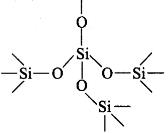(b) Silicon has 3d-orbitals in the valence shell and thus expands its octet giving sp3d2 hybridisation while d-orbitals are not present in the valence shell of carbon. It can undergo ip3-hybridisation only. Thus, carbon is unable to form CF62- anion.

Q.28. The +1 oxidation state in group 13 and +2 oxidation state in group 14 becomes more and more stable with increasing atomic number. Explain.
Ans.
In group 13 and 14, as we move down the group, the tendency of s-electrons of the valence shell to participate in bond formation decreases. This is due to ineffective shielding of s-electrons of the valence shell by the intervening d- and f-electrons. This is called inert pair effect.
Due to this, s-electrons of the valence shell of group 13 and 14 are unable to participate in bonding. Hence, +1 and +2 oxidation states, in group 13 and 14 respectively, become -more stable with increasing atomic number.

Q.29. Carbon and silicon both belong to the group 14, but in spite of the stoichiometric similarity, the dioxides, (i.e., carbon dioxide and silicon dioxide), differ in their structures. Comment.
Ans.
Carbon, the first member of group 14 possesses a pronounced ability to form stable p-p multiple bonds with itself and with other first row elements such as nitrogen and oxygen. In CO2, both the oxygen atoms are linked with carbon atom by double bonds.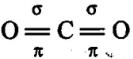However, silicon shows its reluctance in forming p-p multiple bonding due to large atomic size. Thus, in SiO2, oxygen atoms are linked to silicon atom by single covalent bonds giving three dimensional network.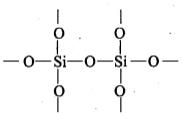Q.30. If a trivalent atom replaces a few silicon atoms in three dimensional network of silicon dioxide, what would be the type of charge on overall structure?
Ans.
If a few tetrahedral Si atoms in a three dimensional network structure of SiO2 are replaced by an equal number of trivalent atoms, then one valence electron of each Si atom will become free. As a result, each substitution of Si atom by a trivalent atom introduces one unit negative charge into the three dimensional network structure of SiO2. Hence, SiO2 becomes negatively charged.

Q.31. When BCl3 is treated with water, it hydrolyses and forms [B[OH]4] only whereas AlCl3 in acidified aqueous solution forms [Al(H2O)6]3+ ion. Explain what is the hybridisation of boron and aluminium in these species?
Ans.
BCl3 + 3H2O→ B(OH)3 + 3HCl
B(OH)3 + H2O →[B(OH)4] + H+
B(OH)3 due to its incomplete octet accepts an electron pair (as OH) to give [B(OH)4]. Boron in this ion involves one 2s orbital and three 2p orbitals. Thus, hybridisation of B in [B(OH)4] is sp3.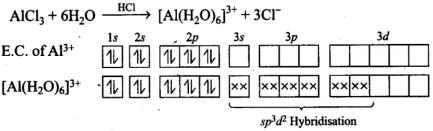Hence, hydridisation of Al is sp3d2.

Q.32. Aluminium dissolves in mineral acids and aqueous alkalies and thus shows amphoteric character. A piece of aluminium foil is treated with dilute hydrochloric acid or dilute sodium hydroxide solution in a test tube and on bringing a burning matchstick near the mouth of the test tube, a pop sound indicates the evolution of hydrogen gas. The same activity when performed with concentrated nitric acid, reaction doesn’t proceed. Explain the reason.
Ans.
Al being amphoteric dissolves both in acids and alkalies evolving H2 gas which bums with a pop sound.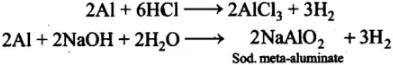With conc. HNO3, Al becomes passive and the reaction does not proceed. This passivity is due to the formation 0f a thin protective layer of its oxide (Al2O3) on the surface of the metal which prevents further action.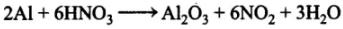Q.33. Explain the following :
(i) Gallium has higher ionisation enthalpy than aluminium.
(ii) Boron does not exist  as B3+ ion.
(iii) Aluminium forms [AlF6]3 ion but boron does not form [BF6]3 ion.
(iv) PbX2 is more stable than PbX4.
(v) Pb4+ acts as an oxidising agent but Sn2+ acts as a reducing agent.
(vi) Electron gain enthalpy of chlorine is more negative as compared to fluorine.
(vii) Tl (NO3)3 acts as an oxidising agent.

(viii) Carbon shows catenation property but lead does not.
(ix) BF3 does not hydrolyse.
(x) Why does the element silicon, not form a graphite like structure whereas carbon does.
Ans. (i) The ionization enthalpy value of Ga is higher than Al due to inability of d- and f- electrons, which have screening effect, to compensate the increase in nuclear charge.
(ii) Boron has small size and sum of ΔiH1 + ΔiH2 + ΔiH3 is very high. Boron does not form B3+ ion therefore, give covalent compounds.
(iii) Al has vacant ‘d orbitals and can expand its co-ordination no. and forms [AlF6]3-. On the other hand, Boron does not have ‘d orbitals and cannot form [BF6]3- and cannot expand its covalence beyond 4 and thus, gives [BF4]-.
(iv) Due to inert pair effect, +2 oxidation state is more stable than +4 oxidation state.
(v) Pb4+ by gaining 2 electrons changes into Pb2+ which is more stable due to inert pair effect. Sn2+ is less stable than Sn4+ by losing electrons. Therefore, Pb4+ acts as an oxidizing agent while Sn2+ acts as a reducing agent.
(vi) The size of F atom is very small and incoming electrons feel interelectronic repulsion and electron gain enthalpy of F is less negative as compared to Cl.
(vii) Due to inert pair effect, Tl is more stable in +1 oxidation state than that of +3 oxidation state. Therefore, Tl (NO3)acts as strong oxidizing agent.
(viii) Carbon atoms have the tendency to link with one another through covalent bonds to form chains and rings. This property is called catenation. This is because C—C bonds are very strong. Down the group the size increases and electronegativity decreases, and, thereby, tendency to show catenation decreases. This can be clearly seen from bond enthalpies values. The order of catenation is C > > Si > Ge Sn. Lead does not show catenation.
(ix) BF3 does not hydrolyze completely. It forms boric acid and fluoroboric acid this is because the HF formed reacts with H3BO3
BF3 + 3H20 → H3BO+ 3HF} x 4
H3BO+ 3HF → H+[BF4]+ 3H2O} x 3
4 BF+ 3H20 → H3BO+ 3[BF4]+ 3H+
(x) In graphite, carbon is sp2 hybridized. Carbon has a tendency to form multiple pπ -pπ bonds due to its small size and highest electronegativity in group 14. Silicon due to its large size and less electronegativity cannot form multiple bonds. Thus, silicon cannot form graphite like structure.

Q.34. Identify the compounds A, X and Z in the following reactions :
(i)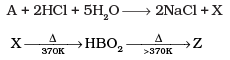Ans. (i) Na2B4O7(A) + 2HCl + 5H2O → 2NaCl + 4B(OH)3(X)
H3BO3(X)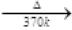HBO2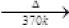B2O3(Z)
Na2B4O7(A), H3BO3(X) and B2O3(Z)

Q.35. Complete the following chemical equations :
Z + 3LiAlH→ X + 3LiF + 3AlF3
X + 6H2O → Y + 6H2
3X + 3O2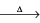B2O3 + 3H2O
Ans
. 4BF+ 3LiAlH4 → 2B2H+ 3LiF + 3A1F3
B2H6(g) + 6H2O(l) → 2B(OH)3(aq) + 6H2(g)
B2H6 + 3O2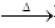B2O+ 3H2O
Z = BF3, X = B2Hand Y = B(OH)or H3BO3

MATCHING TYPE

In the following questions more than one correlation is possible between options of Column I and Column II. Make as many correlations as you can.
Q.36. Match the species given in Column I with the properties mentioned in Column II.

 Column I Column II (i) BF-4 (a) Oxidation state of central atom is +4 (ii) AlCl3 (b) Strong oxidising agent (iii) SnO (c) Lewis acid (iv) PbO2 (d) Can be further oxidised (e) Tetrahedral shape

Ans. (i→e); (ii → c); (iii —> d); (iv →a, b)
(i) BF4 : Tetrahedral shape, sp3 hybridisation, regular geometry.
(ii) AlCl3: Octet of A1 not complete, acts as Lewis acid.
(iii) SnO: Sn2+ can show +4 oxidation state.
(iv) PbO2: Oxidation.state of Pb in PbO2 is +4. Due to inert pair effect, Pb4+ is less stable than Pb2+ and hence acts as strong oxidising agent.

Q.37. Match the species given in Column I with properties given in Column II.

 Column I Column II (i) Diborane (a) Used as a flux for soldering metals (ii) Galluim (b) Crystalline form of silica (iii) Borax (c) Banana bonds (iv) Aluminosilicate (d) Low melting, high boiling, useful for measuring high temperatures (v) Quartz (e) Used as catalyst in petrochemical industries

Ans. (i → c); (ii → d); (iii → a); (iv → e); (v → b)

• BH3 is unstable, forms diborane B2H6 by 3 centre -2 electron bonds, shows banana bonds.
• Gallium with low melting point and high boiling point makes it useful to measure high temperatures.
• Borax is used as a flux for soldering metals.
• Aluminosilicate is used as catalyst in petrochemical industries.
• Quartz, is a crystalline form of silica

Q.38. Match the species given in Column I with the hybridisation given in Column II.

 Column I Column II (i) Boron in [B(OH)4]– (a) sp2 (ii) Aluminium in [Al(H2O)6]3+ (b) sp3 (iii) Boron in B2H6 (c) sp3d2 (iv) Carbon in Buckminsterfullerene (v) Silicon in SiO44– (vi) Germanium in [GeCl6]2–

Ans. (i →b); (ii→ c); (iii → b); (iv→ a); (v →b); (vi→ c)

• Boron in [B(OH)4] is sp3
• Aluminium in [A1(H2O)6]3+ is sp3d2 hybridised
• Boron in B2H6 is sp3
• Carbon in Buckminsterfullerene sp2 is hybridised.
• Silicon in SiO44- is sp3
• Germanium in [GeCl6]2- is sp3d2

ASSERTION AND REASON TYPE QUESTIONS

In the following questions a statement of Assertion (A) followed by a statement of Reason (R) is given. Choose the correct option out of the choices given below each question.
Q.39. Assertion (A): If aluminium atoms replace a few silicon atoms in three dimensional network of silicon dioxide, the overall structure acquires a negative charge.

Reason (R) : Aluminium is trivalent while silicon is tetravalent.
(1) Both A and R are correct and R is the correct explanation of A.
(2) Both A and R are correct but R is not the correct explanation of A.
(3) Both A and R are not correct
(4) A is not correct but R is correct.
Ans. (1) In aluminosilicates (anion), some of the silicon atoms are replaced by aluminium. Since, aluminium is trivalent while silicon is tetravalent, hence we get negatively charged ion.

Q.40. Assertion (A): Silicons are water repelling in nature.
Reason (R) : Silicons are organosilicon polymers, which have (–R2SiO–) as repeating unit.
(1) A  and R both are correct and R is the correct explanation of A.
(2) Both A and R are correct but R is not the correct explanation of A.
(3) A and R both are not true.
(4) A is not true but R is true.

Ans. (2) Silicones are organosilicon polymers and they are hydrophobic in nature. Silicones neither react nor absorb water molecules.

Long Answer Type

Q.41. Describe the general trends in the following properties of the elements in Groups 13 and 14.
(1) Atomic size
(2) Ionisation enthalpy
(3) Metallic character
(4) Oxidation states
(5) Nature of halides
Ans.
Group 13
(1) Atomic size: On moving down the group the size of atom increases because for each successive member of the group one extra shell of electrons is added but in some cases deviation is seen. The size of Ga is less than that of Al due to the poor shielding effect of 10 electrons present in d orbital.
(2) lonization Enthalpy: The first ionization enthalpy of group 14 members is higher than the corresponding members of group 13. The influence of inner core electrons is visible here also. In general, the ionization enthalpy decreases down the group. Small decrease in DiH from Si to Ge to Sn and slight increase in DiH from Sn to Pb is the consequence of poor shielding effect of intervening d and f orbitals and increase in size of the atom.
(3) Metallic character: In group 14, on moving down the metallic character increases. Carbon is non-metal, Si and Ge are metalloid and Sn and Pb are metals.
(4) Oxidation states: The common oxidation states exhibited by these elements are +4 and +2. Carbon also exhibits negative oxidation states. Since, the sum of the first four ionization enthalpies is very high, compounds in +4 oxidation state are generally covalent in nature. In heavier members, the tendency to show +2 oxidation state increases in the sequence Ge < Sn < Pb. It is due to the inability of nselectrons of valence shell to participate in bonding. The relative stabilities of these two oxidation states vary down the group. Carbon and silicon mostly show +4 oxidation state. Germanium forms stable compounds in +4 state and only few compounds in +2 state.
(5) Nature of Halides: These elements can form halides of formula MX2 and MX(X = F, Cl,Br, I). Except carbon, all other members react directly with halogen under suitable conditions to make halides. Most of the compounds (MX4) are covalent in nature. The central metal atom in these halides undergoes sp3 hybridization and the molecule is tetrahedral in shape. Exceptions are SnFand PbF1, which are ionic in nature. PbIdoes not exist because Pb—I bond initially formed during the reaction does not release enough energy to unpair 6selectrons and excite one of them to higher orbital to have four unpaired electrons around lead atom. Heavier members Ge to Pb are able to make halides of formula MX2.

Q.42. Account for the following observations:
(1) AlCl3 is a Lewis acid
(2) Though fluorine is more electronegative than chlorine yet BF3 is a weaker Lewis acid than BCl3
(3) PbO2 is a stronger oxidising agent than SnO2
(4) The +1 oxidation state of thallium is more stable than its +3 state.

Ans. (i) In AlCl3, the octet of Al is incomplete as it has 6 electrons and accepts pair of electrons. Electron accepters are Lewis acids.
(ii) In BF3, boron has a vacant 2p orbital and fluorine has one of the 2p orbital completely filled and unutilized. Both have same energy and can overlap effectively to give pπ—pπ bond. This type of bonding called back bonding. Back bonding is strong in F and decreases down the Gp 17 as the size of halogens increases. Thrust of electrons of Boron is going to be fulfilled by back bonding.
(iii) It is more stable and acts as oxidizing agent. On moving down, a group, inert pair effect increases and it is very strong in case of Pb therefore, PbOis stronger oxidizing agent.
(iv) It is due to inert pair effect.

Q.43. When aqueous solution of borax is acidified with hydrochloric acid, a white crystalline solid is formed which is soapy to touch. Is this solid acidic or basic in nature? Explain.

Ans. An aqueous solution of borax is acidified with HCl to give boric acid.
Na2B4O+ 2HCl + 5H2O → 2NaCl + 4H3BO3
Boric acid is a weak monobasic acid. It is not a protonic acid but acts as a Lewis acid by accepting electrons from a hydroxyl ion:
B(OH)+ 2HOH → [B(OH)4]+ H3O+

Q.44. Three pairs of compounds are given below. Identify that compound in each of the pairs which has group 13 element in more stable oxidation state. Give reason for your choice. State the nature of bonding also.
(i) TlCl3, TlCl
(ii) AlCl3 , AlCl
(iii) InCl3, InCl

Ans. (i) TlCl is more stable than TlCl3 because moving down the group lower oxidation state is more stable due to inert pair effect.
(ii) AlCl3 is more stable because it does not show inert pair effect. It is a covalent compound and acts as a Lewis acid.
(iii) InCl is more stable due to inert pair effect and lower oxidation state +1 is more stable. In shows both the oxidation states +3 and +1.

Q.45. BCl3 exists as monomer whereas AlCl3 is dimerised through halogen bridging.
Give reason. Explain the structure of the dimer of AlClalso.
Ans.
BCl3 does not dimerize because of its small size. BCl3 do not exist as a dimer. Boron cannot accommodate four large sized chloride ions. AlCl3 exist as a dimer in which Al uses its vacant 3p-orbital by coordinate with Cl to complete their octet by forming dimer. AlCl3 achieves stability by forming a dimer.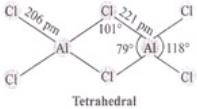Q.46. Boron fluoride exists as BF3 but boron hydride doesn’t exist as BH3. Give reason. In which form does it exist? Explain its structure.
Ans.
In BF3, lone pair of fluorine gives back support of electrons to boron atom pπ—pπ back bonding). This delocalization of electrons reduces the deficiency of electrons and thus reduces Lewis acidic character and increases stability of BF3. In BH3, there is no lone pair of electrons on H atom, therefore, BH3 dimerizes to give B2H6. 4 terminal H atoms and 2 boron atoms lie in one plane and above and below the plane there are two bridging H atoms.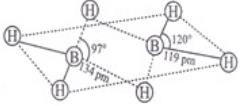Q.47. (1) What are silicones? State the uses of silicones.
(2) What are boranes? Give chemical equation for the preparation of diborane.
Ans.
(1) Silicones area group of organosilicon polymers, which have (R2SiO) as a repeating unit. The starting materials for the manufacture of silicones are alkyl or aryl substituted silicon chlorides, RnSiCi(4-n) where R is alkyl or aryl group. When methyl chloride reacts with silicon in the presence of copper as a catalyst at a temperature 573K various types of methyl substituted chloramine of formula MeSiCl3, Me2SiCl, Me3SiCl with small amount of Me4Si are formed. Hydrolysis of dimethyldichlorosilane, (CH3)2SiCl2

followed by condensation polymerization yields straight chain polymers.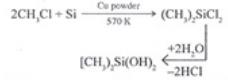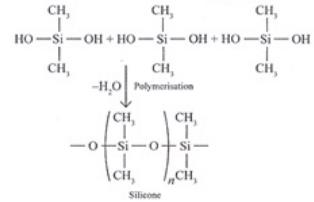Uses: They are used as sealant, greases, electrical insulators and for water proofing of fabrics. Being biocompatible they are also used in surgical and cosmetic plants. (ii) Boranes are the binary compounds of boron and hydrogen like a Alkanes. These are covalent hydrides of formula B2H6 called diborane.
Preparation of diborane:
4BF3 + 3LiAlH4 → 2B2H+ 3LiF + 3A1F3

Q.48. A compound (A) of boron reacts with NMe3 to give an adduct (B) which on hydrolysis gives a compound (C) and hydrogen gas. Compound (C) is an acid. Identify the compounds A, B and C. Give the reactions involved.
Ans.
Compound [A] of boron reacts with NMe3 and gives an adduct [B] thus compound [A] is Lewis acid. Since [B] on hydrolysis gives an acid [C] and H2 gas, therefore [A] is B2H, [B] is an adduct 2BH3NMeand [C] is boric acid. Reactions are as follows:
B2H6(A) + 2NMe3 → 2BH3NMe3(B)
BH3NMe+ 3H20 → H3BO3(C) + NMe+ 6H2

Q.49. A nonmetallic element of group 13, used in making bullet proof vests is extremely hard solid of black colour. It can exist in many allotropic forms and has unusually high melting point. Its trifluoride acts as Lewis acid towards ammonia. The element exihibits maximum covalency of four. Identify the element and write the reaction of its trifluoride with ammonia. Explain why does the trifluoride act as a Lewis acid.
Ans.
In group 13, boron is only non-metallic and extremely hard and also used for making bullet proof vests. Boron exists in many allotropic forms. It usually shows high melting point and does not have ‘d orbital. It can show maximum covalence of 4 by using 2s and 2p orbitals. In trivalent halides ofboron, octet of boron is not completed hence acts as Lewis acid. It reacts with Lewis base and forms adduct.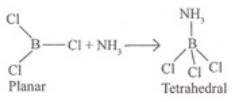Q.50. A tetravalent element forms monoxide and dioxide with oxygen. When air is passed over heated element (1273 K), producer gas is obtained. Monoxide of the element is a powerful reducing agent and reduces ferric oxide to iron. Identify the element and write formulas of its monoxide and dioxide. Write chemical equations for the formation of producer gas and reduction of ferric oxide with the monoxide.
Ans.
Producer gas is a mixture of CO(g) and N2 (g). Carbon is tetravalent and gives monoxide (CO) and dioxide (CO2) with oxygen.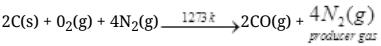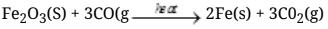Offer running on EduRev: Apply code STAYHOME200 to get INR 200 off on our premium plan EduRev Infinity!

291 docs

,

,

,

,

,

,

,

,

,

,

,

,

,

,

,

,

,

,

,

,

,

;